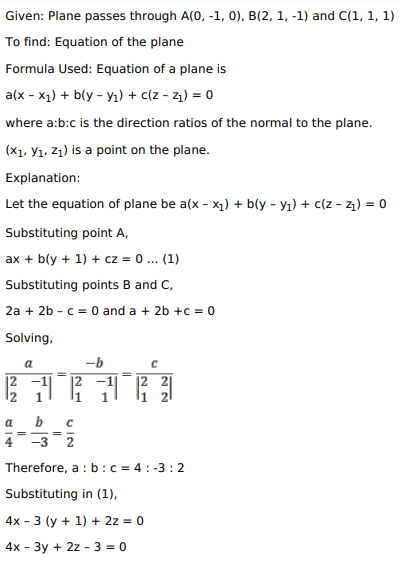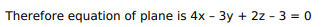# Mark against the correct answer in each of the following:

Question:

Mark against the correct answer in each of the following:

The equation of the plane passing through the points $A(0,-1,0), B(2,1,-1)$ and $C(1,1,1)$ is given by

A. $4 x+3 y-2 z-3=0$

B. $4 x-3 y+2 z+3=0$

C. $4 x-3 y+2 z-3=0$

D. None of these

Solution: